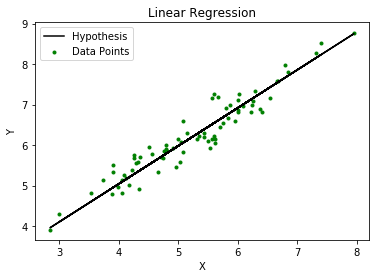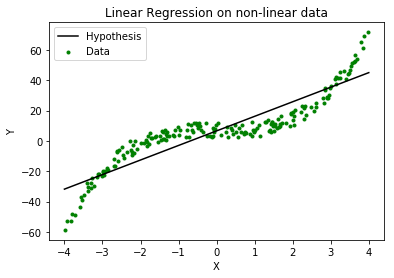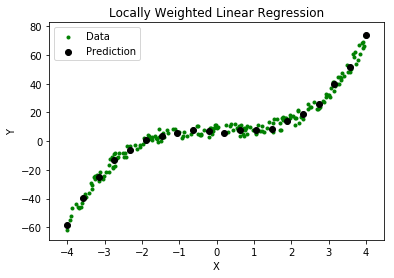# Locally weighted Linear Regression

Linear regression is a supervised learning algorithm used for computing linear relationships between input (X) and output (Y).

The steps involved in ordinary linear regression are:

Training phase: Computeto minimize the cost.Predict output: for given query point,As evident from the image below, this algorithm cannot be used for making predictions when there exists a non-linear relationship between X and Y. In such cases, locally weighted linear regression is used.### Locally Weighted Linear Regression:

Locally weighted linear regression is a non-parametric algorithm, that is, the model does not learn a fixed set of parameters as is done in ordinary linear regression. Rather parametersare computed individually for each query point. While computing, a higher “preference” is given to the points in the training set lying in the vicinity ofthan the points lying far away from.

The modified cost function is:where,is a non-negative “weight” associated with training point.
Fors lying closer to the query point, the value ofis large, while fors lying far away fromthe value ofis small.

A typical choice ofis:where,is called the bandwidth parameter and controls the rate at whichfalls with distance fromClearly, ifis smallis close to 1 and ifis largeis close to 0.

Thus, the training-set-points lying closer to the query pointcontribute more to the costthan the points lying far away from.

For example –

Consider a query point= 5.0 and letandbe two points in the training set such that= 4.9 and= 3.0.
Using the formulawith= 0.5:Thus, the weights fall exponentially as the distance betweenandincreases and so does the contribution of error in prediction forto the cost.

Consequently, while computing, we focus more on reducingfor the points lying closer to the query point (having larger value of).Steps involved in locally weighted linear regression are:

Computeto minimize the cost.Predict Output: for given query point,Points to remember:

• Locally weighted linear regression is a supervised learning algorithm.
• It a non-parametric algorithm.
• There exists No training phase. All the work is done during the testing phase/while making predictions.

# Special 95% discount

## 2000+ Applied Machine Learning & Data Science Recipes

### Portfolio Projects for Aspiring Data Scientists: Tabular Text & Image Data Analytics as well as Time Series Forecasting in Python & R## Two Machine Learning Fields

There are two sides to machine learning:

• Practical Machine Learning:This is about querying databases, cleaning data, writing scripts to transform data and gluing algorithm and libraries together and writing custom code to squeeze reliable answers from data to satisfy difficult and ill defined questions. It’s the mess of reality.
• Theoretical Machine Learning: This is about math and abstraction and idealized scenarios and limits and beauty and informing what is possible. It is a whole lot neater and cleaner and removed from the mess of reality.

Data Science Resources: Data Science Recipes and Applied Machine Learning Recipes

Introduction to Applied Machine Learning & Data Science for Beginners, Business Analysts, Students, Researchers and Freelancers with Python & R Codes @ Western Australian Center for Applied Machine Learning & Data Science (WACAMLDS) !!!

Latest end-to-end Learn by Coding Recipes in Project-Based Learning:

Applied Statistics with R for Beginners and Business Professionals

Data Science and Machine Learning Projects in Python: Tabular Data Analytics

Data Science and Machine Learning Projects in R: Tabular Data Analytics

Python Machine Learning & Data Science Recipes: Learn by Coding

R Machine Learning & Data Science Recipes: Learn by Coding

Comparing Different Machine Learning Algorithms in Python for Classification (FREE)

`Disclaimer: The information and code presented within this recipe/tutorial is only for educational and coaching purposes for beginners and developers. Anyone can practice and apply the recipe/tutorial presented here, but the reader is taking full responsibility for his/her actions. The author (content curator) of this recipe (code / program) has made every effort to ensure the accuracy of the information was correct at time of publication. The author (content curator) does not assume and hereby disclaims any liability to any party for any loss, damage, or disruption caused by errors or omissions, whether such errors or omissions result from accident, negligence, or any other cause. The information presented here could also be found in public knowledge domains.  `